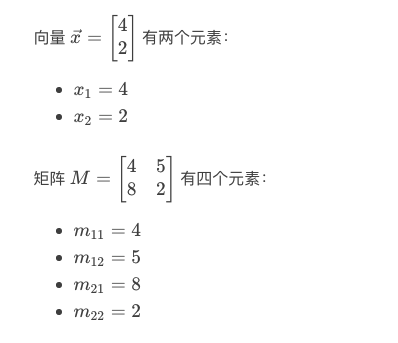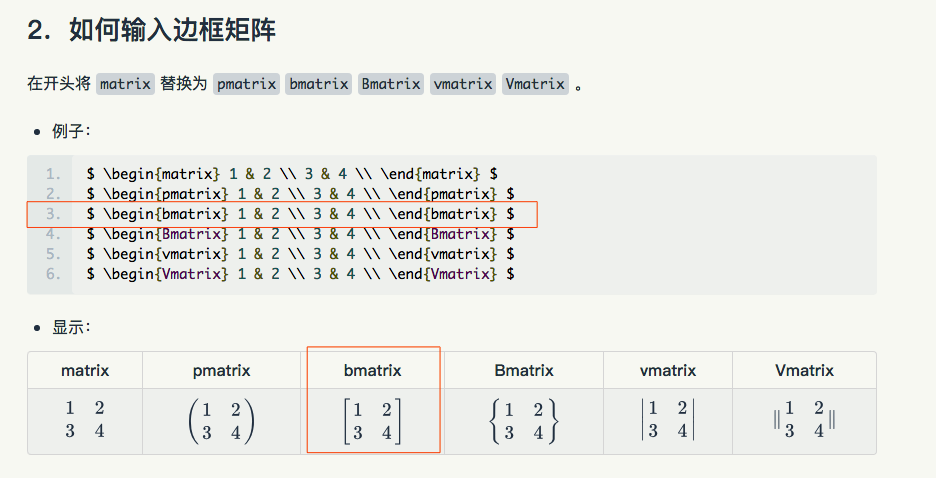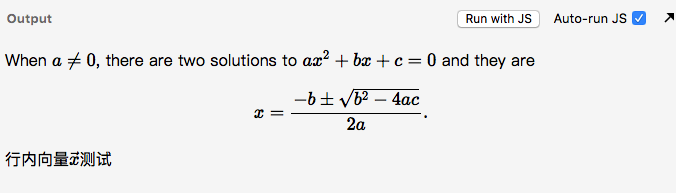# Python:线性代数-基础 (四十)

## LaTex使用

（当然，我们会在这节课详细讲解这些术语）（注意，方程的输入顺序很重要）x_2=2

m_{12}=5

m_{21}=8

## markdown不解析LaTeX语法问题

http://www.mathjax.org/

<script type="text/javascript" async
src="https://cdnjs.cloudflare.com/ajax/libs/mathjax/2.7.5/MathJax.js?config=TeX-MML-AM_CHTML">
</script>

The default math delimiters are $$...$$and $...$for displayed mathematics, and$...$ for in-line mathematics.
Note in particular that the $...$ in-line delimiters are not used by default.That is because dollar signs appear too often in non-mathematical settings, which could cause some text to be treated as mathematics unexpectedly.

https://docs.mathjax.org/en/latest/start.html

 // 这种 $数学公式$ 默认在mathjax插件中不能用，请使用下面的分隔符
$数学公式$   // html 可以直接使用
\$数学公式\$  // 在markdown中使用，必须对反斜杠再做转义，防止被转义取消掉反斜杠\$\vec{x} \$  = \$\begin{bmatrix} a \\\\ b \\\\ c \\\\ \end{bmatrix} \$

 $$数学公式$$

### 使用示例：

example.html

<!DOCTYPE html>
<html>
<meta charset="utf-8">
<meta name="viewport" content="width=device-width">
<title>MathJax example</title>

<body>
<p>
When $a \ne 0$, there are two solutions to $ax^2 + bx + c = 0$ and they are
$$x = {-b \pm \sqrt{b^2-4ac} \over 2a}.$$

</p>
行内向量$\vec{x}$测试

</body>
<script type="text/javascript" async
src="https://cdnjs.cloudflare.com/ajax/libs/mathjax/2.7.5/latest.js?config=TeX-MML-AM_CHTML" async>
</script>
</html>